## Monday, 16 April 2012

### Class 10 - Science - Ch1 - Chemical Reactions & Equations

Ch1 - Chemical Reactions & Equations
MCQs, Q & A, NCERT Solutions

Q1:  A chemical reaction involves in
a. Only breaking of bonds
b. Only formation of bonds
c. Both breaking and formation of bonds
d. None of these

Answer: (c)  Both breaking and formation of bonds

Q2: A balanced chemical equation always obeys
a. Law of conservation of mass
b. Law of thermal equilibrium
c. Law of conservation of energy
d. All of the above

Answer: (a) law of conservation of mass.

Q3: Single displacement reaction involves:
a. Oxidation
b. Reduction
c. Redox
d. Heating

Q4: String of ants, bees contain
a. Formic acid
b. Vinegar
c. Succinic acid
d. Common Salt (NaCl)

Answer: (a) Formic acid

Q5: Some stale food gives a bad taste and a bad smell because of:
a. Corrosion
b. Displacement
c. Heating
d. Rancidity

Q6: A red brown gas is released on heating lead nitrate. It is an example of
a. Combination reaction
b. Oxidation reaction
c. Decomposition reaction
d. Reduction reaction

Q7: The sign ↓ indicates
a. release of gas
b. dissolution of gas
c. formation of a precipitate
d. lowering of temperature

Answer: (c) formation of a precipitate

Q8: Why do gold and silver do not corrode?
Answer:It is because they are less reactive.

Q9: What do you mean by balanced chemical equation?

Answer: An equation that has equal number of atoms of each element on both the sides of equation is called balanced chemical equation, i.e., mass of the reactants is equal to mass of the products.
e.g., 2Mg + O2 → 2MgO

Q10: Define rancidity.
Answer: When fats and oils are oxidized, they become rancid and their smell and taste
change. This process is known as rancidity.

Q11 (CBSE 2007): Write the type of reactions in the following:
i. Reaction between an acid and a base
ii. Rusting of iron

Answer: i. Neutralization reaction
ii. Oxidation reaction

Q12: Give an example of decomposition reaction where energy is supplied in form of light.
sunlight
2AgBr(s) ------------------------> 2Ag (s) + Br2(g)
(Silver Bromide)

Q13: Why does copper vessel acquire green coating in rainy season?
Answer: It reacts with CO2 in the atmosphere and forms a layer of basic Copper Carbonate.

Q14: What is the name of the gas which burns with a pop sound?

Q15: Why is Hydrogen peroxide stored in coloured bottles?
Answer: It is done to prevent photolytic decomposition of hydrogen peroxide.

Q16: Name the oxidizing agent and reducing agent in the following equation.
3MnO2(s) + 4Al(s)   →     3Mn(s)  + 2Al2O3(s)
OR
Name a reducing agent that may be used to obtain manganese from manganese dioxide.

Answer: Reducing agent   : Al
Oxidation Agent : MnO2
Q17: Give two example from everyday life situations where redox reactions are taking place.

Answer: Corrosion and Rancidity

Q18: In electrolysis of water, why is the volume of gas collected over one electrode double that of gas collected over the other electrode ?

Answer: In water (H2O), hydrogen and oxygen are present in the raito of 2:1 by volume.

Q19: Why should a magnesium ribbon be cleaned before burning in air?

Answer: Magnesium is a reactive metal. It combines with oxygen of air to form a layer of magnesium oxide on its surface. Hence, it should be cleaned with a sand paper before burning to remove the oxide layer formed on its surface.

Q20: Explain how respiration is an exothermic reaction.

Answer: During digestion, food is broken down into simpler substances. For example, rice, potatoes and bread contain carbohydrates. These carbohydrates are broken down to form glucose. This glucose combines with oxygen in the cells of our body and provides energy. Hence, respiration is an exothermic process.
C6H12O6(aq) + 6O2(aq) 6CO2(aq) + 6H2O(l) + energy
(Glucouse)

Q21: What factors influence the rate of chemical reaction?

Answer: Following are the factors that can influence the rate of reaction:
• Nature of Reactants. e.g. Mg reacts faster in HCl than in acetic acid.
• Concentration of reactants
• Surface Area of reactants. E.g. Powdered CaCO3 reacts quickly than marble chips.
• Temperature
• Catalyst

Q22: Define Endothermic reaction.

Answer: The reactions in which heat is absorbed are called endothermic reactions. In such reactions heat is shown as one of the reactants. If exact amount of heat absorbed is known then this amount is written otherwise simply the word heat is  written.
E.g. N2(g) + 2O2(g) + heat 2NO(g)
nitric oxide

Q23: A silver ware is kept in a solution of CuSO4 (aq). What change do you expect?
Answer: No reaction. because Silver is less reactive than copper.

Q24(CBSE exam): Name one metal when placed in ferrous sulphate solution will discharge its green colour. Write a chemical equation and state the reasons also.

Answer: Potassium(K) is one example. K is more reactive than iron and will replace it.
2K + FeSO4  →  K2SO4 + Fe
Potassium +  Iron Sulphate Potassium Sulphate + Iron

Q25: Why do we apply paint on iron articles?
Answer: Iron articles are painted to prevent them from rusting. Rusting is oxidation of iron in the presence of air and moisture. The paint layer cuts off the the contact of iron articles from moisture and air.

Q26(CBSE): A solution of a substance ''X'' is used for white washing
(i) Name the substance ''X'' and write its formula
(ii) Write the reaction of the substance ''X'' named in (i) above with water.

(i) The substance ''X'' used for white washing is quick lime (calcium oxide). Its formula is CaO.
(ii) When quick lime is mixed with water, the following reaction takes place:

CaO (s)     +  H2O(l)   →  Ca(OH)2(aq)
Quick Lime           Water                    Slaked Lime
(Calcium Oxide)                          (Calcium Hydroxide)

The white suspension of slaked lime when applied on the walls, combines with carbon dioxide (in air) forming a thin shining layer of calcium carbonate.

Ca(OH)2(aq)  + CO2(g) →   CaCO3(s)  +   H2O(l)
Slaked Lime                                                     Calcium Carbonate

Q27(CBSE): Why is it important to balance a chemical equation?
Answer: The balancing of chemical equation is done to satisfy the law of conservation of mass, i.e. "total mass of all the products of reaction in a chemical reaction is equal the total mass of all the reactants".

Q28: What are the coefficients of the correctly balanced equation?
? Fe2O3 + ? CO ? Fe + ? CO2
(a) 0, 2, 2, 3
(b) 1, 3, 2, 3
(c) 1, 2, 2, 2
(d) 2, 6, 4, 3

Answer: (b) Ferric Oxide + Carbon Mono-oxide →  Iron + Carbon DiOxide

Q29: What are the coefficients of the correctly balanced equation?
? BaCl2 + ? Al2(SO4)3   → ? BaSO4 + ? AlCl3 (aq)
(a)  1, 1, 1, 2
(b)  3, 2, 3, 2
(c)  3, 1, 3,2
(d)  2, 1, 1, 2

Answer: (c) 3 BaCl2 + 1 Al2(SO4)3   → 3 BaSO4 + 2 AlCl3 (aq)

Barium Chloride + Aluminium Sulphate → Barium Sulphate + Aluminium Chloride

Q30(CBSE2011): What is the colour of FeSO4.7H2O crystals ? How does this colour change upon heating ? Give balanced chemical equation for the changes.

Answer: Hydrated ferrous sulphate crystals are green in colour. On heating, single reactant breaks down to give simpler products. This is a decomposition reaction.

Ferrous sulphate crystals (FeSO4.7H2O) lose water when heated and the colour of the crystals changes. It then decomposes to ferric oxide (Fe2O3), sulphur dioxide (SO2) and sulphur trioxide (SO3). Ferric oxide is a solid, while SO2 and SO3 are gases.

You may use Wolfram Chemical Reaction Calculator E.g. Type lime + Water --> Calcium Hydroxide

Q31: Quick like (CaO - Calcium Oxide) reaction with water is regarded as exothermic. A student mixes these two products in a test tube and touches its side surface. Which of the following statement correctly describes the student's observation?

(a) The test tube becomes cold due to release of heat energy.
(b) The test tube becomes hot due to release of heat energy.
(c) The test tube becomes hot due to absorption of heat energy.
(d) The test tube becomes cold due to absorption of heat energy.

Answer:  (b) The test tube becomes hot due to release of heat energy.

Q32: The decomposition of vegetable matter into compost is an example of _____.
(Choose the correct option).
(a) endothermic reaction.
(b) exothermic reaction.

Q33(CBSE): Write a balanced chemical equation to represent the following reaction:
Iron reacts with steam to form Iron(II,III) oxide and hydrogen gas.

` 3Fe(s) + 4H2O(l)  ▬▬▬▬▬▶ Fe3O4(s) + 4H2(g)`
` Iron     Water           Iron Oxide  Hydrogen `

Q34(CBSE): Identify the substance oxidized, substance reduced, oxidising agent and reducing agent.
MnO2 + 4HCl MnCl2 + 2H2O + Cl2

Substance Oxidised: HCl
Substance reduced: MnO2
Reducing Agent: HCl
Oxidising Agent: MnO2

Q35: Bromine gas (Br2) reacts with Sodium Carbonate (Na2CO3) in aqueous solution and gives sodium bromide (NaBr), sodium bromate (NaBrO3) and carbon-dioxide gas. How many number of sodium bromide molecules obtained in the balanced chemical equation.

Answer: From the question, we infer the unbalanced chemical equation is:
Br2 + Na2CO3  →  NaBr  + NaBrO3  + CO2

Balanced equation is:
3 Br2 + 3 Na2CO3  →  5 NaaBr  + NaBrO3  + 3 CO2
It means 5 molecules of NaBr are obtained.

Q36: A numismatist (coin collector) has been collecting gold coins, silver coins and copper coins for a long time. One day he observed a black coating on silver coins and green coating on copper coins. What chemical process is responsible for these coatings. Also name the chemical formula of the black and green coatings.

Answer: The coating occurred due to a chemical process called corrosion. Black coating is due to deposit of Silver Sulphide (Ag2S). Green coating is due to formation of Copper Carbonate (CuCO3)
(Note: Silver usually is corrosion resistant and does not oxidize. However presence of sulphur gases in atmosphere can react and cause sulphur-corrosion. )

Q37: Methane gas (CH4) when burns with oxygen (O2) gives carbon dioxide and water. Write the balanced chemical equation and provide the following information in terms of reactants and products in the equation.
(a) no. of molecules
(b) no. of moles
(c) molar mass
(d) total mass of reactants and products
What does statement (d) infer?

Answer:  The balanced chemical equation is:
CH4 (g) + 2 O2(g)  2H2O (l) + CO2(g)
Methane  + Oxygen water + carbon di-oxide

ReactantsProducts
a. No. of moldeculesCH4 (g), O2(g)   H2O, CO2(g)
b. no. of moles1 mole of  CH4+ 1 mole of  O2(g)2 moles of H2O + 1 mol of  CO2
c. molar mass16g of CH4+ 64g of O2 44g of H2O + 36g of CO2
d.total mass80g 80g

Statement (d) infers that law of conservation of mass is obeyed.1.TELL US ALL D NAMES OF CHEMICAL FORMULAS

1.Please see post http://cbse-notes.blogspot.in/2012/05/nice-online-calculators.html.
It has chemical formula online widget.

2.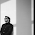3.whoooo its tooo nice man Really I loved It

4.its is used to solve my probelms

5.WHY DO FIREFLIES GLOW AT NIGHT?

1.There are biological and chemical reasons for it. This biochemical process is called bio-luminescence and is due to the reaction of oxygen with a chemical protein called luciferin. Glowing at night, perhaps the reasons are biological i.e. attracting their partners. The best explanation I found at Scientific American

6.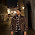very very very good questions......satisfied myself by answering these

7.how to print it

1.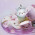8.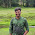I think there is a mistake in answer given in question number 35. That is carbon dioxide is present in product side, but in answer it is CO3. I don't think carbon can show such a valency. When we are balancing, it is 9 oxygen in reactent side whereas 12 in product side. Is it a mistake or my fault ?
Any way the blog is very very helpful. Congrats...

9.good...!!!!!!!! usefull 4 board x am's........

10.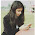This comment has been removed by the author.

11.12.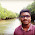I need all the chemical formulas .......:)

13.Gud...

14.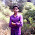15.1.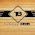By taking screenshot

16.This comment has been removed by a blog administrator.

17.i liked the site for my extra questions thnkssssssssss......... a lottttttttttt

18.why after ripening mangoes turn green to yellow????????........

1.Usually raw fruits are green due to presence of chlorophyll pigment. Ripening is a biochemical process due to presence of enzymes which reduces the green pigment and breaks down pectin cell wall and other complex substances (complex sugars). It leads to turning fruits green to yellow.

19.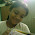very usefull

20.very usefull

21.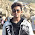22.This comment has been removed by the author.

23.i want to ask that energy in the form of underground pressure and heat is required to convert vegetable waste into compost which is endothermic then how is it an exothermic reaction? Q-32

1.Generally decomposition reactions are endothermic but not always. Decomposition of vegetation by bacteria is a slow oxidation process which is exothermic.

24.Nice guys
One must read it
Thanks

25.26.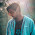27.Nice for exam

28.Nice for exam

29.plz tell me ten names of acid with their chemical names ph value and their chemical formula